# Package Modelica.​MathLibrary of mathematical functions (e.g., sin, cos) and of functions operating on vectors and matrices

### Information

This package contains basic mathematical functions (such as sin(..)), as well as functions operating on vectors, matrices, nonlinear functions, and Boolean vectors.

#### Main Authors

Martin Otter and Marcus Baur
Deutsches Zentrum für Luft- und Raumfahrt e.V. (DLR)
Institut für Systemdynamik und Regelungstechnik (DLR-SR)
Forschungszentrum Oberpfaffenhofen
D-82234 Wessling
Germany
email: Martin.Otter@dlr.de

Extends from `Modelica.​Icons.​Package` (Icon for standard packages).

### Package Contents

NameDescription
`acos`Inverse cosine (-1 <= u <= 1)
`acosh`Inverse of cosh (area hyperbolic cosine)
`asin`Inverse sine (-1 <= u <= 1)
`asinh`Inverse of sinh (area hyperbolic sine)
`atan`Inverse tangent
`atan2`Four quadrant inverse tangent
`atan3`Four quadrant inverse tangent (select solution that is closest to given angle y0)
`baseIcon1`This icon will be removed in future Modelica versions, use Modelica.Math.Icons.AxisLeft instead.
`baseIcon2`This icon will be removed in future Modelica versions, use Modelica.Math.Icons.AxisCenter instead.
`BooleanVectors`Library of functions operating on Boolean vectors
`cos`Cosine
`cosh`Hyperbolic cosine
`Distributions`Library of distribution functions
`exp`Exponential, base e
`FastFourierTransform`Library of functions for the Fast Fourier Transform (FFT)
`Icons`Icons for Math
`isEqual`Determine if two Real scalars are numerically identical
`isPowerOf2`Determine if the integer input is a power of 2
`log`Natural (base e) logarithm (u shall be > 0)
`log10`Base 10 logarithm (u shall be > 0)
`Matrices`Library of functions operating on matrices
`Nonlinear`Library of functions operating on nonlinear equations
`Random`Library of functions for generating random numbers
`sin`Sine
`sinh`Hyperbolic sine
`Special`Library of special mathematical functions
`tan`Tangent (u shall not be -pi/2, pi/2, 3*pi/2, ...)
`tanh`Hyperbolic tangent
`tempInterpol1`Temporary function for linear interpolation (will be removed)
`tempInterpol2`Temporary function for vectorized linear interpolation (will be removed)
`Vectors`Library of functions operating on vectors
`wrapAngle`Wrap angle to interval ]-pi,pi] or [0,2*pi[

## Function Modelica.​Math.​isEqualDetermine if two Real scalars are numerically identical

### Information

#### Syntax

```Math.isEqual(s1, s2);
Math.isEqual(s1, s2, eps=0);
```

#### Description

The function call "`Math.isEqual(s1, s2)`" returns true, if the two Real scalars s1 and s2 are identical. Otherwise the function returns false. The equality check is performed by "abs(s1-s2) ≤ eps", where "eps" can be provided as third argument of the function. Default is "eps = 0".

#### Example

```  Real s1 = 2.0;
Real s2 = 2.0;
Real s3 = 2.000001;
Boolean result;
algorithm
result := Math.isEqual(s1,s2);     // = true
result := Math.isEqual(s1,s3);     // = false
result := Math.isEqual(s1,s3,0.1); // = true
```

Extends from `Modelica.​Icons.​Function` (Icon for functions).

### Inputs

TypeNameDescription
`Real``s1`First scalar
`Real``s2`Second scalar
`Real``eps`The two scalars are identical if abs(s1-s2) <= eps

### Outputs

TypeNameDescription
`Boolean``result`= true, if scalars are identical

## Function Modelica.​Math.​isPowerOf2Determine if the integer input is a power of 2

### Information

#### Syntax

```Math.isPowerOf2(i);
```

#### Description

The function call "`Math.isPowerOf2(i)`" returns true, if the Integer input i is a power of 2. Otherwise the function returns false. The Integer input has to be >=1.

#### Example

```  Integer i1 = 1;
Integer i2 = 4;
Integer i3 = 9;
Boolean result;
algorithm
result := Math.isPowerOf2(i1);     // = true 2^0
result := Math.isPowerOf2(i2);     // = true 2^2
result := Math.isPowerOf2(i3);     // = false
```

Extends from `Modelica.​Icons.​Function` (Icon for functions).

### Inputs

TypeNameDescription
`Integer``i`Integer scalar

### Outputs

TypeNameDescription
`Boolean``result`= true, if integer scalar is a power of 2

## Function Modelica.​Math.​sinSine

### Information

This function returns y = sin(u), with -∞ < u < ∞: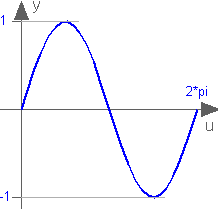Extends from `Modelica.​Math.​Icons.​AxisLeft` (Basic icon for mathematical function with y-axis on left side).

### Inputs

TypeNameDescription
`Angle``u`

### Outputs

TypeNameDescription
`Real``y`

## Function Modelica.​Math.​cosCosine

### Information

This function returns y = cos(u), with -∞ < u < ∞: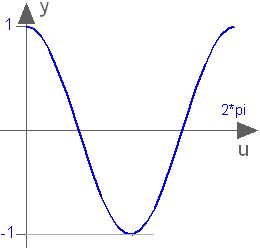Extends from `Modelica.​Math.​Icons.​AxisLeft` (Basic icon for mathematical function with y-axis on left side).

### Inputs

TypeNameDescription
`Angle``u`

### Outputs

TypeNameDescription
`Real``y`

## Function Modelica.​Math.​tanTangent (u shall not be -pi/2, pi/2, 3*pi/2, ...)

### Information

This function returns y = tan(u), with -∞ < u < ∞ (if u is a multiple of (2n-1)*pi/2, y = tan(u) is +/- infinity).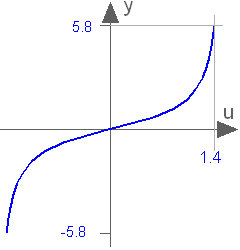Extends from `Modelica.​Math.​Icons.​AxisCenter` (Basic icon for mathematical function with y-axis in the center).

### Inputs

TypeNameDescription
`Angle``u`

### Outputs

TypeNameDescription
`Real``y`

## Function Modelica.​Math.​asinInverse sine (-1 <= u <= 1)

### Information

This function returns y = asin(u), with -1 ≤ u ≤ +1: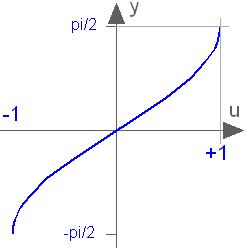Extends from `Modelica.​Math.​Icons.​AxisCenter` (Basic icon for mathematical function with y-axis in the center).

### Inputs

TypeNameDescription
`Real``u`

### Outputs

TypeNameDescription
`Angle``y`

## Function Modelica.​Math.​acosInverse cosine (-1 <= u <= 1)

### Information

This function returns y = acos(u), with -1 ≤ u ≤ +1: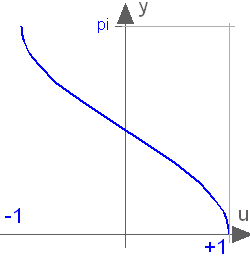Extends from `Modelica.​Math.​Icons.​AxisCenter` (Basic icon for mathematical function with y-axis in the center).

### Inputs

TypeNameDescription
`Real``u`

### Outputs

TypeNameDescription
`Angle``y`

## Function Modelica.​Math.​atanInverse tangent

### Information

This function returns y = atan(u), with -∞ < u < ∞: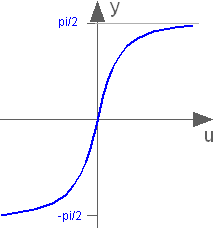Extends from `Modelica.​Math.​Icons.​AxisCenter` (Basic icon for mathematical function with y-axis in the center).

### Inputs

TypeNameDescription
`Real``u`

### Outputs

TypeNameDescription
`Angle``y`

## Function Modelica.​Math.​atan2Four quadrant inverse tangent

### Information

This function returns y = atan2(u1,u2) such that tan(y) = u1/u2 and y is in the range -pi < y ≤ pi. u2 may be zero, provided u1 is not zero. Usually u1, u2 is provided in such a form that u1 = sin(y) and u2 = cos(y):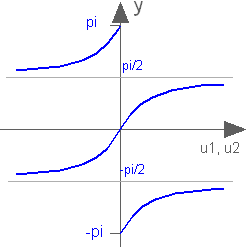Extends from `Modelica.​Math.​Icons.​AxisCenter` (Basic icon for mathematical function with y-axis in the center).

### Inputs

TypeNameDescription
`Real``u1`
`Real``u2`

### Outputs

TypeNameDescription
`Angle``y`

## Function Modelica.​Math.​atan3Four quadrant inverse tangent (select solution that is closest to given angle y0)

### Information

This function returns y = atan3(u1,u2,y0) such that tan(y) = u1/u2 and y is in the range: -pi < y-y0 ≤ pi.
u2 may be zero, provided u1 is not zero. The difference to Modelica.Math.atan2(..) is the optional third argument y0 that allows to specify which of the infinite many solutions shall be returned: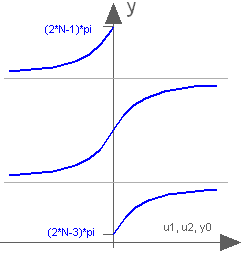Note, for the default case (y0=0), exactly the same result as with atan2(..) is returned.

Extends from `Modelica.​Math.​Icons.​AxisCenter` (Basic icon for mathematical function with y-axis in the center).

### Inputs

TypeNameDescription
`Real``u1`
`Real``u2`
`Angle``y0`y shall be in the range: -pi < y-y0 <= pi

### Outputs

TypeNameDescription
`Angle``y`

## Function Modelica.​Math.​sinhHyperbolic sine

### Information

This function returns y = sinh(u), with -∞ < u < ∞: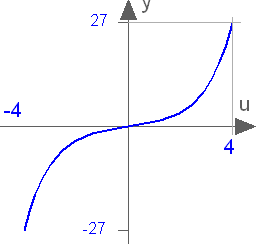Extends from `Modelica.​Math.​Icons.​AxisCenter` (Basic icon for mathematical function with y-axis in the center).

### Inputs

TypeNameDescription
`Real``u`

### Outputs

TypeNameDescription
`Real``y`

## Function Modelica.​Math.​coshHyperbolic cosine

### Information

This function returns y = cosh(u), with -∞ < u < ∞: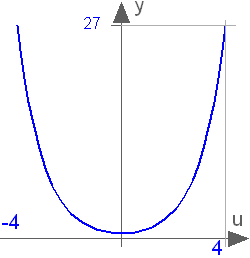Extends from `Modelica.​Math.​Icons.​AxisCenter` (Basic icon for mathematical function with y-axis in the center).

### Inputs

TypeNameDescription
`Real``u`

### Outputs

TypeNameDescription
`Real``y`

## Function Modelica.​Math.​tanhHyperbolic tangent

### Information

This function returns y = tanh(u), with -∞ < u < ∞: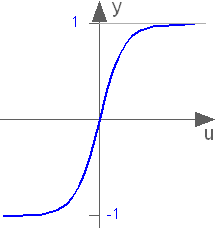Extends from `Modelica.​Math.​Icons.​AxisCenter` (Basic icon for mathematical function with y-axis in the center).

### Inputs

TypeNameDescription
`Real``u`

### Outputs

TypeNameDescription
`Real``y`

## Function Modelica.​Math.​asinhInverse of sinh (area hyperbolic sine)

### Information

The function returns the area hyperbolic sine of its input argument u. This inverse of sinh(..) is unique and there is no restriction on the input argument u of asinh(u) (-∞ < u < ∞):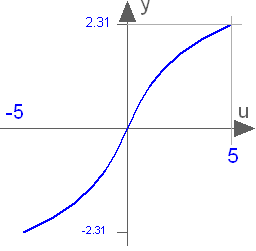Extends from `Modelica.​Math.​Icons.​AxisCenter` (Basic icon for mathematical function with y-axis in the center).

### Inputs

TypeNameDescription
`Real``u`

### Outputs

TypeNameDescription
`Real``y`

## Function Modelica.​Math.​acoshInverse of cosh (area hyperbolic cosine)

### Information

This function returns the area hyperbolic cosine of its input argument u. The valid range of u is

```  +1 ≤ u < +∞
```

If the function is called with u < 1, an error occurs. The function cosh(u) has two inverse functions (the curve looks similar to a sqrt(..) function). acosh(..) returns the inverse that is positive. At u=1, the derivative dy/du is infinite. Therefore, this function should not be used in a model, if u can become close to 1: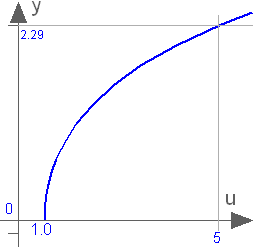Extends from `Modelica.​Math.​Icons.​AxisLeft` (Basic icon for mathematical function with y-axis on left side).

### Inputs

TypeNameDescription
`Real``u`

### Outputs

TypeNameDescription
`Real``y`

## Function Modelica.​Math.​expExponential, base e

### Information

This function returns y = exp(u), with -∞ < u < ∞: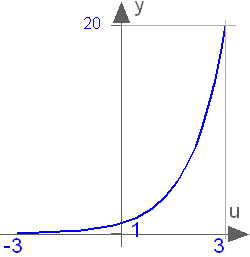Extends from `Modelica.​Math.​Icons.​AxisCenter` (Basic icon for mathematical function with y-axis in the center).

### Inputs

TypeNameDescription
`Real``u`

### Outputs

TypeNameDescription
`Real``y`

## Function Modelica.​Math.​logNatural (base e) logarithm (u shall be > 0)

### Information

This function returns y = log(10) (the natural logarithm of u), with u > 0: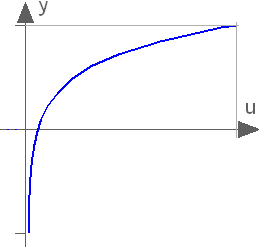Extends from `Modelica.​Math.​Icons.​AxisLeft` (Basic icon for mathematical function with y-axis on left side).

### Inputs

TypeNameDescription
`Real``u`

### Outputs

TypeNameDescription
`Real``y`

## Function Modelica.​Math.​log10Base 10 logarithm (u shall be > 0)

### Information

This function returns y = log10(u), with u > 0: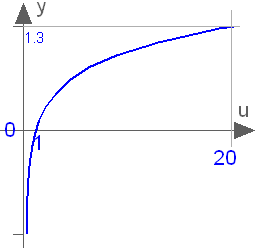Extends from `Modelica.​Math.​Icons.​AxisLeft` (Basic icon for mathematical function with y-axis on left side).

### Inputs

TypeNameDescription
`Real``u`

### Outputs

TypeNameDescription
`Real``y`

## Function Modelica.​Math.​wrapAngleWrap angle to interval ]-pi,pi] or [0,2*pi[

### Information

This function wraps the input angle `u` to the interval ]-pi,pi], if `positiveRange == false`. Otherwise the input angle `u` is wrapped to the interval [0,2*pi[.

Extends from `Modelica.​Math.​Icons.​AxisCenter` (Basic icon for mathematical function with y-axis in the center).

### Inputs

TypeNameDescription
`Angle``u`Input angle
`Boolean``positiveRange`Use only positive output range, if true

### Outputs

TypeNameDescription
`Angle``y`Wrapped output angle

## Partial Function Modelica.​Math.​baseIcon1This icon will be removed in future Modelica versions, use Modelica.Math.Icons.AxisLeft instead.

### Information

This icon will be removed in future versions of the Modelica Standard Library. Instead the icon Modelica.Math.Icons.AxisLeft shall be used.

Extends from `Modelica.​Icons.​ObsoleteModel` (Icon for classes that are obsolete and will be removed in later versions).

## Partial Function Modelica.​Math.​baseIcon2This icon will be removed in future Modelica versions, use Modelica.Math.Icons.AxisCenter instead.

### Information

This icon will be removed in future versions of the Modelica Standard Library. Instead the icon Modelica.Math.Icons.AxisCenter shall be used.

Extends from `Modelica.​Icons.​ObsoleteModel` (Icon for classes that are obsolete and will be removed in later versions).

## Function Modelica.​Math.​tempInterpol1Temporary function for linear interpolation (will be removed)

### Information

Extends from `Modelica.​Icons.​Function` (Icon for functions) and `Modelica.​Icons.​ObsoleteModel` (Icon for classes that are obsolete and will be removed in later versions).

### Inputs

TypeNameDescription
`Real``u`input value (first column of table)
`Real``table[:,:]`table to be interpolated
`Integer``icol`column of table to be interpolated

### Outputs

TypeNameDescription
`Real``y`interpolated input value (icol column of table)

## Function Modelica.​Math.​tempInterpol2Temporary function for vectorized linear interpolation (will be removed)

### Information

Extends from `Modelica.​Icons.​Function` (Icon for functions) and `Modelica.​Icons.​ObsoleteModel` (Icon for classes that are obsolete and will be removed in later versions).

### Inputs

TypeNameDescription
`Real``u`input value (first column of table)
`Real``table[:,:]`table to be interpolated
`Integer``icol[:]`column(s) of table to be interpolated

### Outputs

TypeNameDescription
`Real``y[1,size(icol, 1)]`interpolated input value(s) (column(s) icol of table)

Generated 2018-12-12 12:14:36 EST by MapleSim.